# 排序算法

• 内部排序：将所有数据放在内存中处理，排序时不涉及数据的内外交换
• 外部排序：因数据量太大，内存不能一次容纳全部的排序记录，而是通过磁盘和内存的数据传输才能进行排序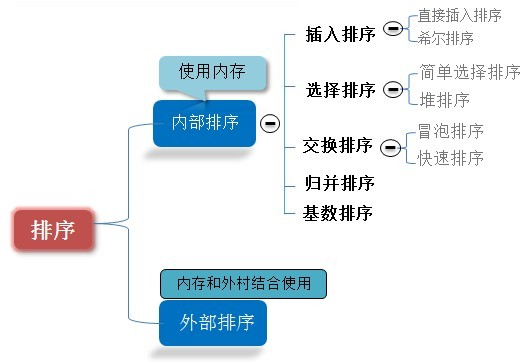• 稳定：如果a原本在b前面，而a=b，排序之后a仍然在b的前面；

• 不稳定：如果a原本在b的前面，而a=b，排序之后a可能会出现在b的后面；

• 时间复杂度： 一个算法执行所耗费的时间。

• 空间复杂度：运行完一个程序所需内存的大小。

• n: 数据规模

• k: “桶”的个数

• In-place: 占用常数内存，不占用额外内存

• Out-place: 占用额外内存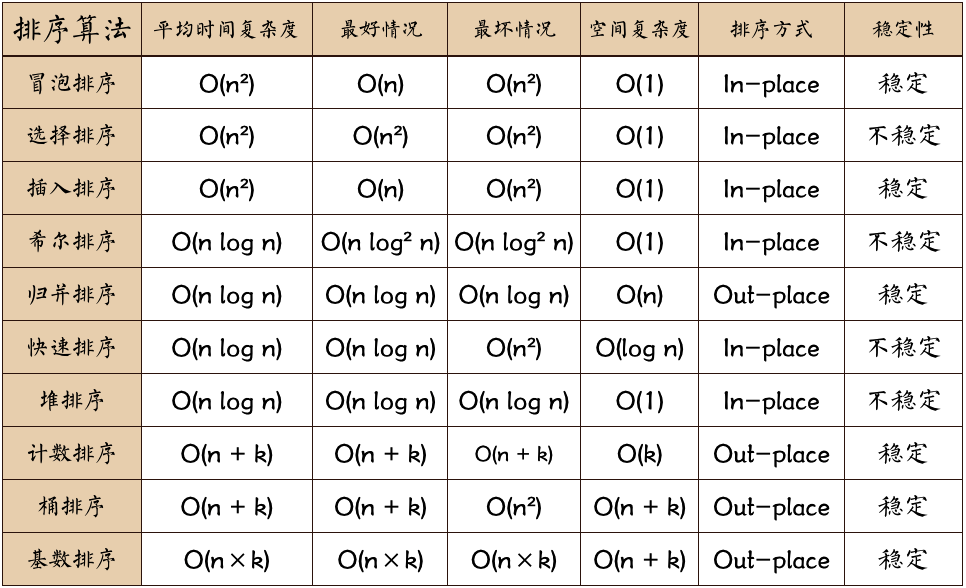# 1、冒泡排序

**排序思想：**两个数比较大小，较大的数下沉，较小的数冒起来。

• 比较相邻的两个数据，如果第一个比第二个大，就交换位置（向右冒泡）。
• 对每一对相邻元素作同样的工作，从开始第一对到结尾的最后一对，这样在最后的元素应该会是最大的数；
• 针对所有的元素重复以上的步骤，除了最后一个；
• 重复步骤1~3，直到排序完成。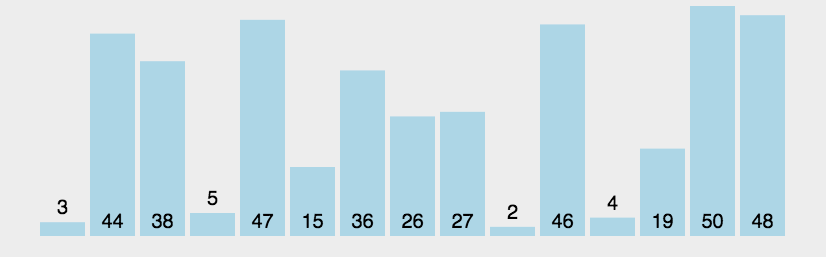O(n2 ) O(n) O(n2 ) O(1)

public static void bubbleSort(int [] arr){
if (arr == null || arr.length <=1) {
return;
}
int temp;//临时变量
for(int i=0; i<arr.length-1; i++){   //表示趟数，一共arr.length-1次。
for(int j=arr.length-1; j>i; j--){
if(arr[j] < arr[j-1]){   // 相邻元素对比，交换
temp = arr[j];
arr[j] = arr[j-1];
arr[j-1] = temp;
}
}
}
}


public class Test {
public static void main(String[] args) {
int[] array = new int[]{10, 20};
System.out.println(Arrays.toString(array));

// 临时变量法
temSwap(array, 0, 1);
System.out.println(Arrays.toString(array));

// 算术法
array = new int[]{10, 20};
arithmeticSwap(array, 0, 1);
System.out.println(Arrays.toString(array));

// 位运算法
array = new int[]{10, 20};
bitSwap(array, 0, 1);
System.out.println(Arrays.toString(array));
}
// 通过临时变量交换数组array的i和j位置的数据
public static void temSwap(int[] array, int i, int j) {
int temp = array[i];
array[i] = array[j];
array[j] = temp;
}

// 通过算术法交换数组array的i和j位置的数据（有可能溢出）
public static void  arithmeticSwap(int[] array, int i, int j) {
array[i] = array[i] + array[j];
array[j] = array[i] - array[j];
array[i] = array[i] - array[j];
}
//通过位运算法交换数组array的i和j位置的数据
// a ^ a =0
// 0 ^ b =b
public static void  bitSwap(int[] array, int i, int j) {
array[i] = array[i]^array[j];
array[j] = array[i]^array[j]; // array[i]^array[j]^array[i]=array[j]
array[i] = array[i]^array[j]; // array[i]^array[j]^array[j]=array[i]
}
}


# 2、选择排序

• 首先在未排序序列中找到最小（大）元素，存放到排序序列的起始位置，
• 然后，再从剩余未排序元素中继续寻找最小（大）元素，然后放到已排序序列的末尾。
• 以此类推，直到所有元素均排序完毕。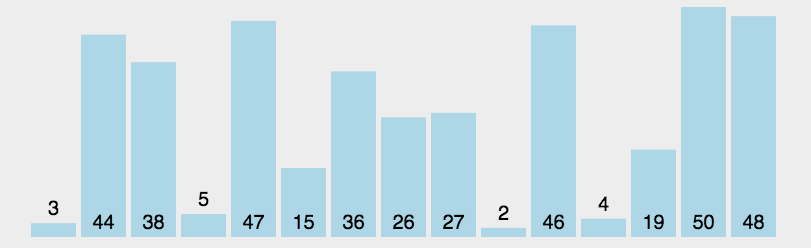public static void selectionSort(int[] array) {
if (array == null || array.length <=1) {
return;
}
int lenth = array.length;
int minIndex, temp;
for (int i = 0; i < lenth - 1; i++) {
minIndex = i;
for (int j = i + 1; j < lenth; j++) {
if (array[j] < array[minIndex]) { // 寻找最小的数
minIndex = j; // 将最小数的索引保存
}
}
if (minIndex != i) {
temp = array[i];
array[i] = array[minIndex];
array[minIndex] = temp;
}
}
}


# 3、插入排序

• 从第一个元素开始，该元素可以认为已经被排序；
• 取出下一个元素，在已经排序的元素序列中从后向前扫描；
• 如果该元素（已排序）大于新元素，将该元素移到下一位置；
• 重复步骤3，直到找到已排序的元素小于或者等于新元素的位置；
• 将新元素插入到该位置后；
• 重复步骤2~5。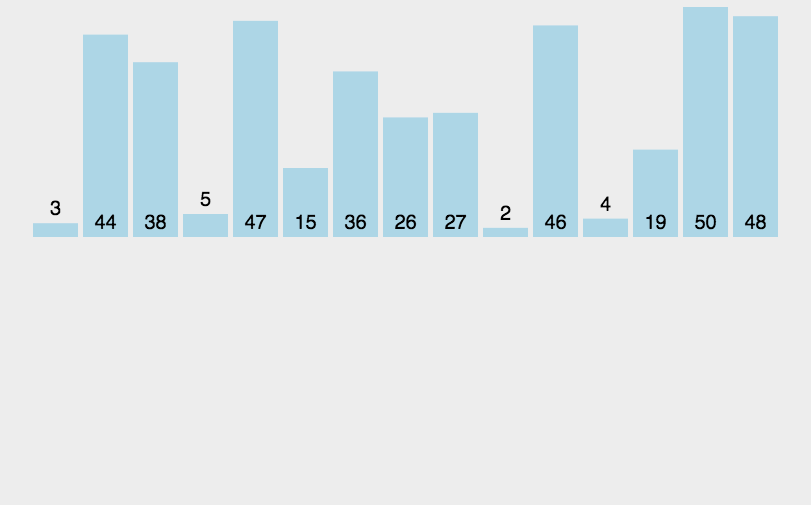public static void insertionSort(int[] array) {
if (array == null || array.length <=1) {
return;
}
int temp;
for (int i = 0; i < array.length - 1; i++) {
for (int j = i + 1; j > 0; j--) {
if (array[j] < array[j - 1]) {
temp = array[j - 1];
array[j - 1] = array[j];
array[j] = temp;
} else { // 不需要交换
break;
}
}
}
}


# 4、希尔排序

• 先取一个小于n 的整数 d1 作为第一个增量，把文件的全部记录分组。
• 所有距离为 d1 的倍数的记录放在同一个组中。先在各组内进行直接插入排序；
• 然后，取第二个增量d2<d1重复上述的分组和排序，直至所取的增量 =1( < …<d2<d1)，即所有记录放在同一组中进行直接插入排序为止。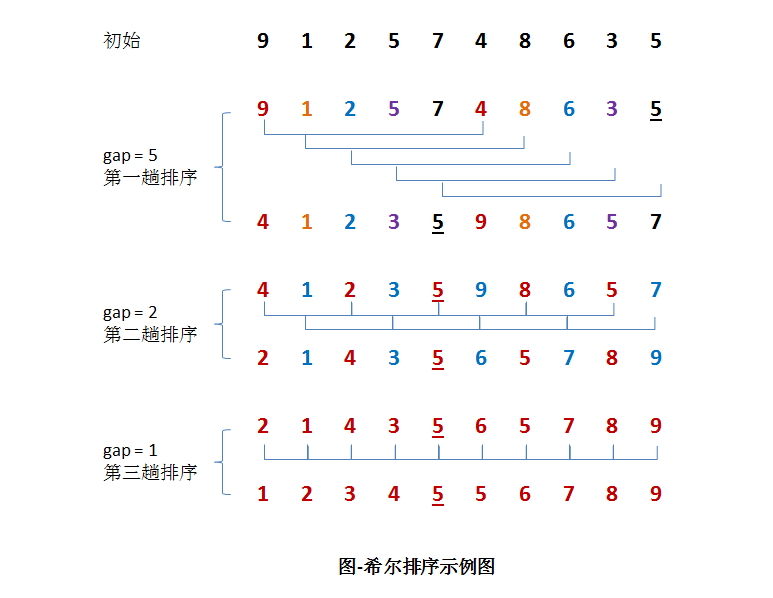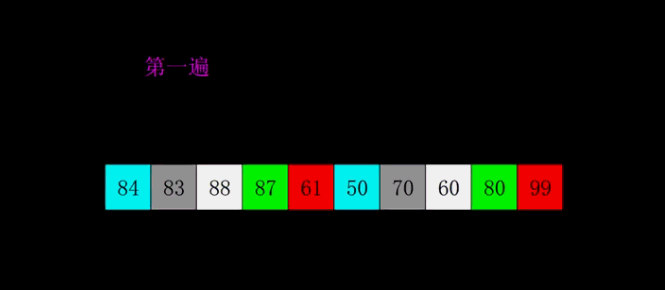public static void shellSort(int[] array) {
if (array == null || array.length <=1) {
return;
}
int temp = 0;
int incre = array.length;  // 间隔或增量

while (incre > 1) {
// 2 相当于每个子序列的个数，首次这里以 length/2 为间隔分组，可以得到 incre 组序列
incre = incre / 2;
for (int k = 0; k < incre; k++) { // 根据增量分为若干子序列

// 每组序列进行 插入排序
// 注意，遍历时每组序列中相邻两个元素之间步长为 incre
for (int i = k + incre; i < array.length; i += incre) {
for (int j = i; j > k; j -= incre) {
if (array[j] < array[j - incre]) {
temp = array[j - incre];
array[j - incre] = array[j];
array[j] = temp;
} else {
break;
}
}
}
}
}
}


# 5、归并排序

• 把长度为n的输入序列分成两个长度为n/2的子序列；
• 对这两个子序列分别采用归并排序；
• 将两个排序好的子序列合并成一个最终的排序序列。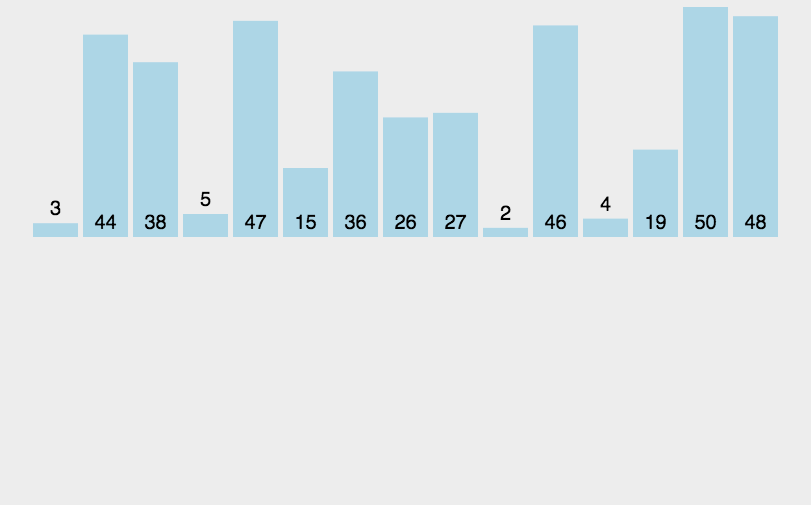public static int[] mergeSort(int[] array) {
if (array == null || array.length <=1) {
return;
}
int num = array.length / 2;
int[] left = Arrays.copyOfRange(array, 0, num);
int[] right = Arrays.copyOfRange(array, num, array.length);

return mergeTwoArray(mergeSort(left), mergeSort(right));
}

private static int[] mergeTwoArray(int[] leftArr, int[] rightArr) {
int i = 0, j = 0, k = 0;
// 申请额外空间保存归并之后数据
int[] result = new int[leftArr.length + rightArr.length];

while (i < leftArr.length && j < rightArr.length) { // 选取两个序列中的较小值放入新数组
if (leftArr[i] <= rightArr[j]) {
result[k++] = leftArr[i++];
} else {
result[k++] = rightArr[j++];
}
}

while (i < leftArr.length) { // 序列a中多余的元素移入新数组
result[k++] = leftArr[i++];
}
while (j < rightArr.length) {// 序列b中多余的元素移入新数组
result[k++] = rightArr[j++];
}
return result;
}


# 6、快速排序

• 从数列中挑出一个元素，称为 “基准”（pivot）；
• 重新排序数列，所有元素比基准值小的摆放在基准前面，所有元素比基准值大的摆在基准的后面（相同的数可以到任一边）。在这个分区退出之后，该基准就处于数列的中间位置。这个称为分区（partition）操作；
• 递归地（recursive）把小于基准值元素的子数列和大于基准值元素的子数列排序。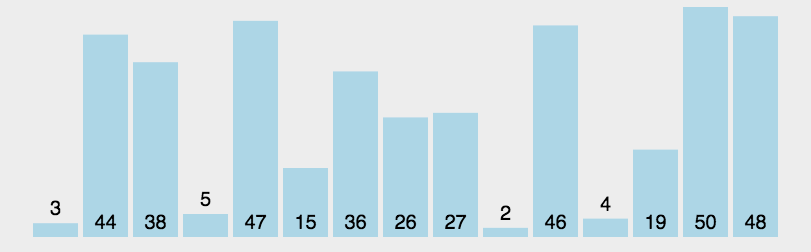public static void quickSort(int arr[], int low, int high) {
if (arr == null || arr.length <= 0) {
return;
}
if (low >= high) {
return;
}

int left = low;
int right = high;
int temp = arr[left]; // 基准的值

while (left < right) {
//从后向前，找到比base基准值小的值
while (left < right && arr[right] >= temp) {
right--;
}
// 从后向前找到比基准小的元素，先进行交换的第2步，因为arr[left] 已经备份到temp 了
arr[left] = arr[right];

//从前往后，找到比base基准值大的值
while (left < right && arr[left] <= temp) {
left++;
}
// 交换这两个数
arr[right] = arr[left]; // 进行交换的第3步
}
arr[left] = temp; // 基准值填补到中间位置，准备分治递归快排
quickSort(arr, low, left - 1);
quickSort(arr, left + 1, high);
}


# 7、堆排序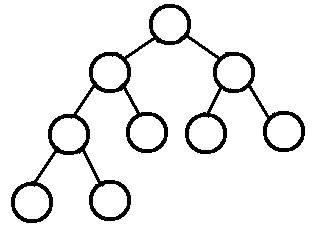)

• 大顶堆：指每个结点的值都大于或等于其左右孩子结点的值
• 小顶堆：每个结点的值都小于或等于其左右孩子结点的值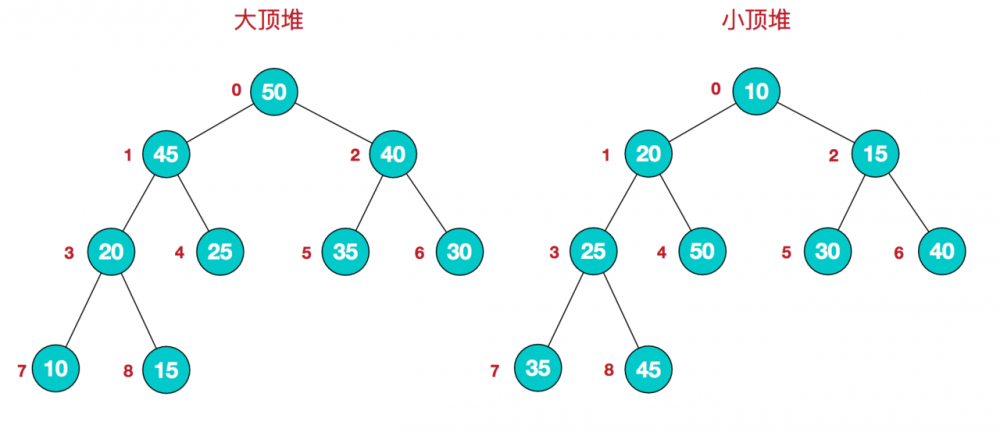)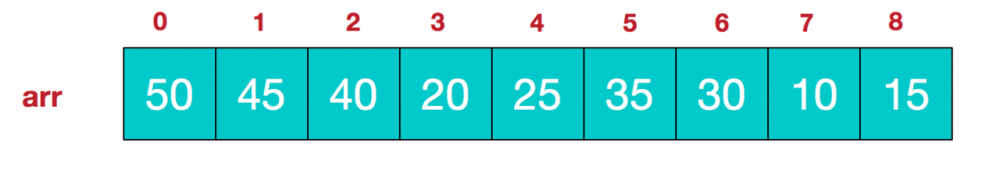大顶堆：arr[i] >= arr[2i+1] && arr[i] >= arr[2i+2]



• 将无需序列构建成一个大顶堆，此堆为初始的无序区；（一般升序采用大顶堆，降序采用小顶堆)。

• 将堆顶元素与末尾元素交换，将最大元素"沉"到数组末端；即R与R[n]交换

• 由于交换后新的堆顶R可能违反堆的性质，重新调整结构，使其满足堆定义，

• 然后继续交换堆顶元素与当前末尾元素，反复执行调整+交换步骤，直到整个序列有序。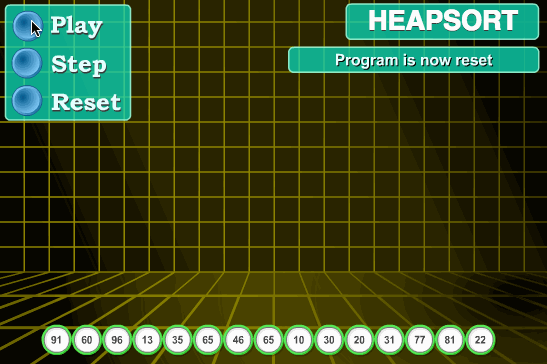public static void heapSort(int[] arr) {
if (arr == null || arr.length <= 0) {
return;
}
// 1.构建大顶堆
for (int i = arr.length / 2 - 1; i >= 0; i--) {
// 从第一个非叶子结点从下至上，从右至左调整结构
}
// 2.调整堆结构+交换堆顶元素与末尾元素
for (int j = arr.length - 1; j > 0; j--) {
//swap(arr, 0, j);// 将堆顶元素与末尾元素进行交换
int temp = arr;
arr = arr[j];
arr[j] = temp;
adjustHeap(arr, 0, j);// 重新对堆进行调整
}

}
private static void adjustHeap(int[] arr, int i, int length) {
int temp = arr[i];// 先取出当前元素i
// 从i结点的左子结点开始，也就是2i+1处开始
for (int k = i * 2 + 1; k < length; k = k * 2 + 1) {
// 如果左子结点小于右子结点，k指向右子结点
if (k + 1 < length && arr[k] < arr[k + 1]) {
k++;
}
// 如果子节点大于父节点，将子节点值赋给父节点（不用进行交换）
if (arr[k] > temp) {
arr[i] = arr[k];
i = k;
} else {
break;
}
}
arr[i] = temp;// 将temp值放到最终的位置
}


# 8、计数排序

• 找出待排序的数组中最大和最小的元素；
• 统计数组中每个值为i的元素出现的次数，存入数组C的第i项；
• 对所有的计数累加（从C中的第一个元素开始，每一项和前一项相加）；
• 反向填充目标数组：将每个元素i放在新数组的第C(i)项，每放一个元素就将C(i)减去1。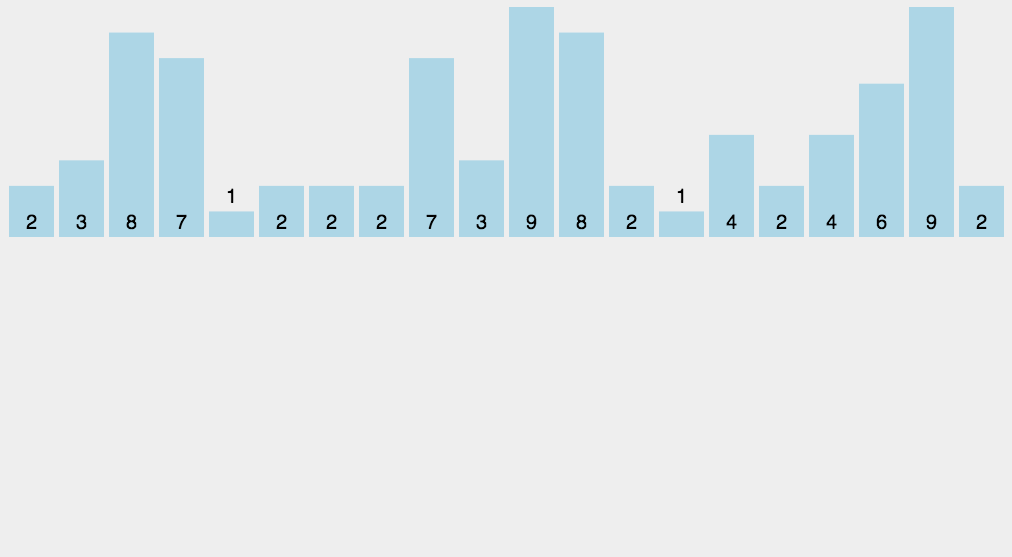public static void sort(int[] arr) {
if (arr == null || arr.length <= 0) {
return;
}
int max = arr,min = arr;
// 计算最大最小值
for (int i : arr) {
if (i > max) {
max = i;
}
if (i < min) {
min = i;
}
}
// Java 8 新写法
//		max = Arrays.stream(arr).max().getAsInt();
//		min = Arrays.stream(arr).min().getAsInt();

int length = arr.length;
int range = max - min + 1; // 最大最小元素之间范围[min, max]的长度

// 1. 计算频率，在需要的数组长度上额外  +1
int[] count = new int[range + 1];
System.out.println("范围[min, max]的长度"+(range + 1));
for (int i = 0; i < length; i++) {
// 使用加1后的索引，有重复的该位置就自增
count[arr[i] - min + 1]++;
}
System.out.println("计算频率："+Arrays.toString(count));

// 2. 频率 -> 元素的开始索引
for (int i = 0; i < range; i++) {
count[i + 1] += count[i];
System.out.println("索引："+Arrays.toString(count));
}

// 3. 元素按照开始索引分类，用到一个和待排数组一样大临时数组存放数据
int[] aux = new int[length];
for (int i = 0; i < length; i++) {
// 填充一个数据后，自增，以便相同的数据可以填到下一个空位
aux[count[arr[i] - min]++] = arr[i];
}
// 4. 数据回写
for (int i = 0; i < length; i++) {
arr[i] = aux[i];
}
}


# 9、桶排序

• 初始化装入连续区间元素的n个桶，每个桶用来装一段区间中的元素。
• 遍历待排序的数据，将其映射到对应的桶中，保证每个桶中的元素都在同一个区间范围中。
• 对每个不是空的桶进行排序；最终将所有桶中排好序的元素连起来。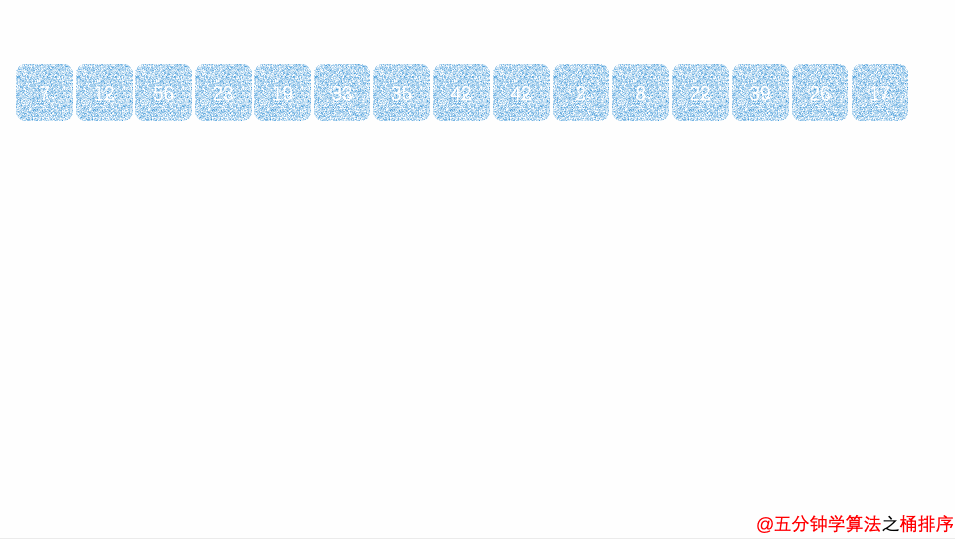public static void bucketSort(int[] arr) {
int bucketSize = arr.length;
if (arr == null || arr.length <= 0) {
return;
}

int minValue = arr;
int maxValue = arr;
for (int i = 1; i < arr.length; i++) {
if (arr[i] < minValue) {
minValue = arr[i];                // 输入数据的最小值
} else if (arr[i] > maxValue) {
maxValue = arr[i];                // 输入数据的最大值
}
}
// Java 8 新写法
//		max = Arrays.stream(arr).max().getAsInt();
//		min = Arrays.stream(arr).min().getAsInt();

int bucketCount = (int) (Math.floor((maxValue - minValue) / bucketSize) + 1);
int[][] buckets = new int[bucketCount];

// 利用映射函数将数据分配到各个桶中
for (int i = 0; i < arr.length; i++) {
// 获取映射索引
int index = (int) Math.floor((arr[i] - minValue) / bucketSize);

//自动扩容，并保存数据
int[] bucket = buckets[index];
bucket = Arrays.copyOf(bucket, bucket.length + 1);
bucket[bucket.length - 1] = arr[i];  // a[i] 放入桶的最后面
buckets[index] = bucket;
}

int arrIndex = 0;
for (int[] bucket : buckets) {
if (bucket.length <= 0) {
continue;
}
// 对每个桶进行排序，这里使用了插入排序
insertionSort(bucket);
for (int value : bucket) {
arr[arrIndex++] = value;
}
}
}


# 10、基数排序

• 取得数组中的最大数，并取得位数；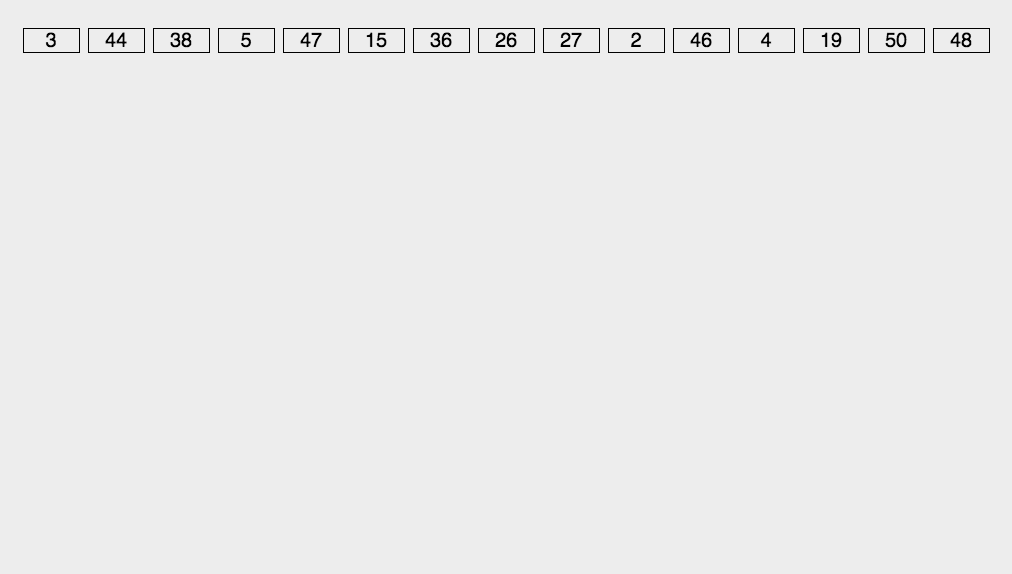public static void radixSort(int[] arr) {
// 获取最大数值
int maxValue = arr;
for (int value : arr) {
if (maxValue < value) {
maxValue = value;
}
}

// 获取最高位数
int maxDigit = 0;
for (long temp = maxValue; temp != 0; temp /= 10) {
maxDigit++;
}

int mod = 10;
int dev = 1;
int digit = 1; // 当前位置值
while (digit <= maxDigit) {
// 数组的第一维表示可能的余数0-9
// 如果要考虑负数的情况，这里可以将队列数扩展一倍，其中 [0-9]对应负数，[10-19]对应正数
int[][] counter = new int;
for (int i = 0; i < arr.length; i++) {
int index = (arr[i] % mod) / dev; // 如果考虑了负数，还需要 +10
// 自动扩容，并保存数据
int[] bucket = counter[index];
bucket = Arrays.copyOf(bucket, bucket.length + 1);
bucket[bucket.length - 1] = arr[i]; // a[i] 放入桶的最后面
counter[index] = bucket;
}
// 重新对按照统一位数值大小排序组
int pos = 0;
for (int[] bucket : counter) {
for (int value : bucket) {
arr[pos++] = value;
}
}
mod *= 10;
dev *= 10;
digit++;
}
}


• 基数排序：根据键值的每位数字来分配桶；
• 计数排序：每个桶只存储单一键值；适用于小范围数排序
• 桶排序：每个桶存储一定范围的数值

# 资料参考